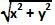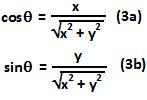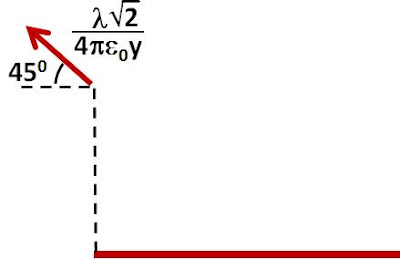## Friday, February 5, 2010

### Irodov Problem 3.14Consider an infinitesimally small section of the thread at a distance x from its one end and of length dx. The charge contained in this infinitesimally small section is given by,The distance of this infinitesimally small section from the point in question (as shown in the figure) is. The electric field due to this infinitesimally small section of the thread is given by,From elementary trigonometry we know that,Hence we have,The direction of the electric field is at 135 degrees to the rod as shown in the figure below.1.it would be a great help if you plz post some theory on the use of vector forms in these problems and how to integrate these vectors

2.This comment has been removed by the author.

3.This comment has been removed by the author.

4.How should one use Gauss' Law in this case?

5.we can use gauss law by completing an imaginary fig and then finding the net charge on it

6.Y to constant hai bhenchod x variable hai, so only integrate x from 0 to infinity

1.abe madarchod denominator ka x bhi to hai.